## Commercial Numerical Analysis and Math Libraries

•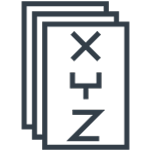• #### Arm Performance Libraries

Arm Performance Libraries are commercially available math libraries from Arm. These libraries include optimized BLAS, LAPACK and FFT.

•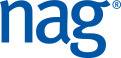• #### NAG Library

A commercially available numerical analysis library from NAG, with a collection of over 1600 routines covering a wide range of mathematical and statistical areas.

## Open Source Math Libraries

• #### OpenBLAS

An optimized BLAS library based on GotoBLAS2 1.13 BSD version.

• #### FFTW

A software library for computing Fast Fourier Transforms (FFT).

• #### BLIS

A software framework for instantiating high-performance BLAS-like dense linear algebra libraries.

• #### ATLAS

A mature open source implementation of BLAS APIs for C and Fortran77 with some routines from LAPACK.

•• #### Eigen

A C++ template library for linear algebra: matrices, vectors, numerical solvers, and related algorithms.

•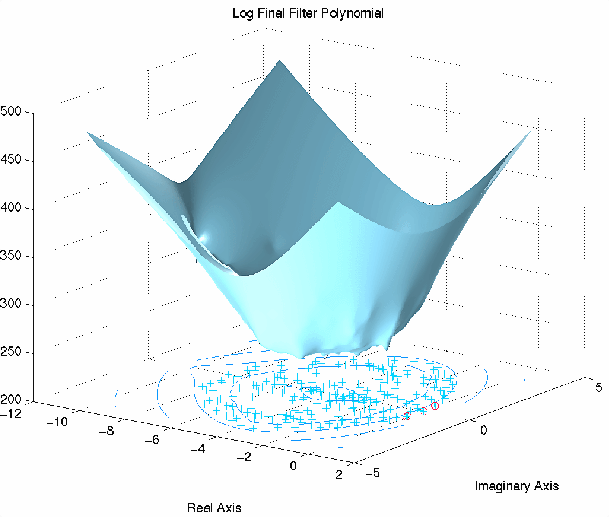• #### ARPACK

A collection of Fortran77 subroutines designed to solve large scale eigenvalue problems.

•• #### GNU Scientific Library

A software library for numerical computations in applied mathematics and science.

•• #### Elemental

An open-source, openly-developed software for distributed memory-dense and sparse-direct linear algebra and optimization.

•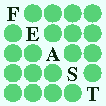• #### FEAST

A free high-performance numerical library for solving the Hermitian and non-Hermitian eigenvalue problems, and obtaining all the eigenvalues and (right/left) eigenvectors within a given search interval or arbitrary domain in the complex plane.

•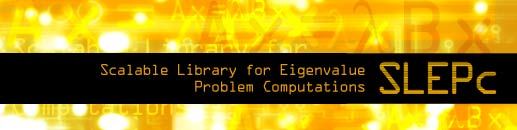• #### SLEPc

A software library for the solution of large scale sparse eigenvalue problems on parallel computers. It is an extension of PETSc and can be used for linear eigenvalue problems in either standard or generalized form, with real or complex arithmetic.

•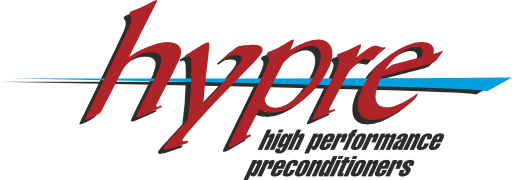• #### Hypre

A software library of high performance preconditioners and solvers for the solution of large, sparse linear systems of equations on massively parallel computers.

•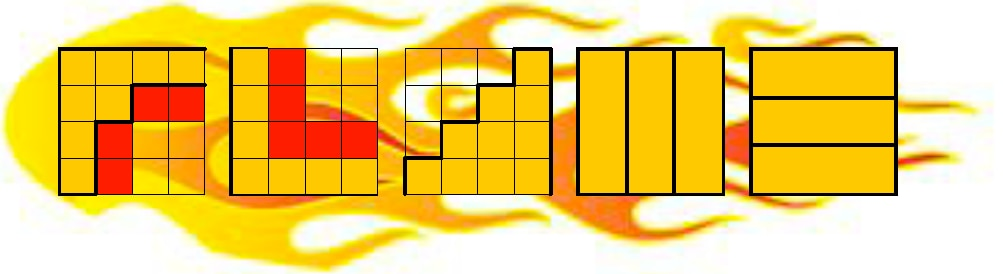• #### FLAME

A high performance dense linear algebra library that is the result of the FLAME methodology for systematically developing dense linear algebra libraries.

• #### PETSc

A suite of data structures and routines for the scalable (parallel) solution of scientific applications modeled by partial differential equation.

•• #### Trilinos

Algorithms and enabling technologies for the solution of large-scale, complex multi-physics engineering, and scientific problems.

•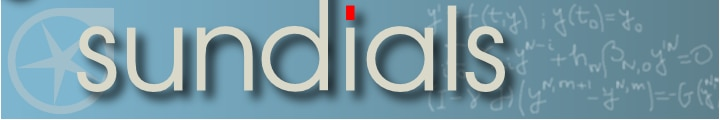• #### SUNDIALS

A suite of nonlinear and differential/algebraic equation solvers.

• #### PBLAS

Parallel Basic Linear Algebra Subprograms

• #### ScaLAPACK

A library of high-performance linear algebra routines for parallel distributed memory machines. ScaLAPACK solves dense and banded linear systems, least squares problems, eigenvalue problems, and singular value problems.

•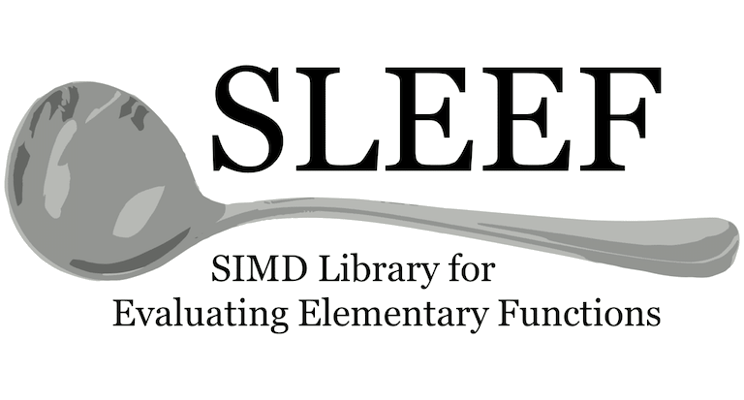• #### SLEEF

A SIMD library that provides vector implementations of all the floating-point math functions defined in the C99 standard.